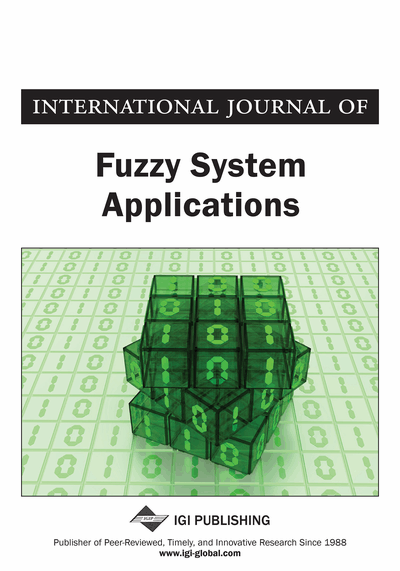# Intuitionistic Fuzzy Possibility Degree Measure for Ordering of IVIFNs with Its Application to MCDM

Animesh Biswas (University of Kalyani, Kalyani, India) and Samir Kumar (University of Kalyani, Kalyani, India)
DOI: 10.4018/IJFSA.2019100101

## Abstract

In this article, the concept of an intuitionistic fuzzy possibility degree (IFPD) for ordering several interval-valued intuitionistic fuzzy numbers (IVIFNs) is introduced. The IFPD ranks IVIFNs by distinguishing the comparable and non-comparable components of the joint intervals of membership and non-membership degrees. The incomparable cases of nested joint intervals can also rank respective IVIFNs through the proposed IFPD approach. An intuitionistic fuzzy possibility preference relation based on the proposed IFPD measure for IVIFNs is defined as a more effective tool for modelling uncertainty than existing intuitionistic preference relations. Further, an approach for solving multicriteria interval-valued intuitionistic fuzzy decision-making problems based on IFPD measure of IVIFNs is advanced also provides a possibility degree as supplementary information to the ranking of alternatives. The validity and effectiveness of the advanced approach are demonstrated through two illustrative examples.
Article Preview
Top

## 1. Introduction

Multi-criteria decision making (MCDM) problems are very complex in nature due to making selections of some courses of action in presence of multiple conflicting attributes. Fuzzy sets (Zadeh, 1965) as a mathematical tool for modelling vagueness and uncertainty (Biswas & Dewan, 2012; Biswas & Modak, 2012, 2013) in real-life situations (Biswas et al., 2011, 2014; Debnath et al., 2016) were generalized to the intuitionistic fuzzy sets (IFSs) (Atanassov, 1986, 1999) by augmenting non-membership function with the membership function and thus IFSs getting more freedom of expression of reality. Atanassov and Gargov (1989) further generalized the concepts of IFSs to the interval-valued IFSs (IVIFSs) for better capturing of uncertainties in nature. Ranking of alternatives happens to be fundamental to every decision-making problem (Nayagam & Sivaraman, 2011; Ye, 2009; Tao et al., 2016). Chen and Tan (1994) proposed score function while Hong and Choi (2000) defined accuracy function for an intuitionistic fuzzy number (IFN) and both these concepts together define total order on the set of IFNs. There are several applications of IFNs in clustering algorithms (Iakovidis et al., 2008; Xu & Wu, 2010; Verma et al., 2016) and decision making (Biswas & De, 2016). Xu (2007b) defined score function and accuracy function for the interval-valued intuitionistic fuzzy number (IVIFN), respectively, as the difference and sum of the midpoints of membership and non-membership interval degrees. Sengupta and Pal (2000) proposed two approaches, acceptability index (optimistic decision maker’s preference index) and fuzzy preference ordering (for pessimistic decision makers) between two interval numbers. The first one based on the midpoints of interval numbers was designed to focus on the grade of acceptability of one interval number to be inferior to the other. The use of midpoints to rank interval numbers limits its acceptability. Wang et al. (2005) developed a preference degree ranking method of interval numbers independent of the midpoints of interval numbers. Wei and Tang (2010) applied the possibility measure (Wang et al., 2005) for interval numbers to define an order on IFNs by transforming an IFN into an interval number by taking membership degree as lower bound and fuzzy complement of non-membership degree as upper bound. Three equivalent possibility degree measures (Facchinetti et al., 1998; Xu & Da, 2002; Wang et al., 2005) for comparing interval numbers have found extensive use in the literature. Wan and Dong (2014) advanced the idea of possibility degree measure for comparing two IVIFNs as the average possibility measure of comparison of respective membership interval numbers and non-membership interval numbers in reverse order without proving transitivity property. Transitivity is a fundamental idea in decision making that is used to check the consistency of making choices by a decision maker and the absence of whose would yield misleading judgment (Xu et al., 2014). Kumar and Biswas (2017) extended the aforesaid three equivalent possibility measures to three other equivalent possibility degree measures for comparing IVIFNs holding weak transitivity property and thus satisfying minimum requirement of consistency. Biswas and Kumar (2018) utilized these possibility measures and presented TOPSIS techniques in interval-valued intuitionistic fuzzy (IVIF) settings employing mathematical programming and normalized hamming distance between IFSs. Tao et al. (2016) ranked interval-valued fuzzy numbers using the idea of IFPD and applied it to fuzzy multi-attribute decision making problems.

## Complete Article List

Search this Journal:
Reset
Open Access Articles: Forthcoming
Volume 9: 4 Issues (2020): Forthcoming, Available for Pre-Order
Volume 8: 4 Issues (2019)
Volume 7: 4 Issues (2018)
Volume 6: 4 Issues (2017)
Volume 5: 4 Issues (2016)
Volume 4: 4 Issues (2015)
Volume 3: 4 Issues (2013)
Volume 2: 4 Issues (2012)
Volume 1: 4 Issues (2011)
View Complete Journal Contents Listing